# Sorting Algorithms Using Python

Hola Readers, welcome back to another post. This one is a quick note on main sorting algorithms and their implementations using Python. The aspects like time complexity, space complexity, stability, recursiveness will also be discussed with respect to each algorithm. The main 5 algorithms discussed in here is:

1. Selection Sort
2. Bubble Sort
3. Insertion Sort
4. Merge Sort
5. Quick Sort

Let's dive right in without any further delay.

# Sorting

Sorting is the mechanism of arranging elements in ascending or descending manner, according to a certain property. To sort a list or collection, they should contain homogenous elements.

If not sorted, to find an element, we have to check each element in the list or collection, one by one (This is known as linear search). In a worst-case scenario, the element won’t exist in the list. Then you have to go through each element in the whole list, just to find out that it does not exist.

Imagining that one comparison takes 1 millisecond (ms), and we have a list with size 2⁶⁴, then the time taken to find out that the element does not exist is 2⁶⁴ milliseconds.

If the list is sorted, we can use a searching algorithm like Binary search (hope you know binary search). In that case, to find an element in the above-mentioned list, we need to make log2 (2⁶⁴) comparisons to find that element does not exist. This means log2 (2⁶⁴) ms i.e. 64 ms.

Sorting algorithms can be classified using the following criteria or aspects of them.

A) Time complexity: Look at the aspect of time taken to do the sorting

B) Space complexity or Memory usage: Looks at the aspect of memory usage during the sorting. According to this classification, there are two types of algorithms;

1. Constant memory (In-place algorithms)
2. Memory usage growing with input size

C) Stability: If it is a stability algorithm, when the element keys (the basis of sorting) are equal, it preserves the order of the original list for the equal keys.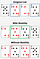Stability in Sorting

D) Internal vs External: Internal means all records are in the main memory or RAM while the external means records reside in disks or tapes as there are a lot.

E) Recursive vs Non-recursive: Recursive means that a method calls itself repeatedly to get the intension achieved. Examples for recursion algorithms include Quick sort, Merge sort etc., while non-recursive algorithms include Insertion sort, Selection sort etc.

Out of these criteria, the main consideration often lies in the Time and Space complexity.

1. Selection Sort
2. Bubble Sort
3. Insertion Sort
4. Merge Sort
5. Quick Sort

# Selection Sort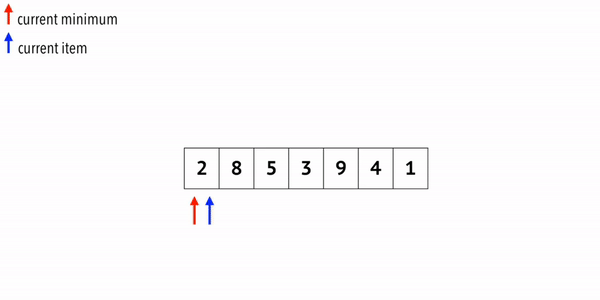Selection Sort (Source: Michael Sambol — Youtube)

This algorithm divides the list into two parts as sorted and unsorted (sorted denoted in light green in above gif).

Starts from the left corner, assuming the first element is the current minimum and the current item. Move current item pointer one step at a time comparing with the current minimum. Update the current minimum pointer if current item pointer encounters a value lesser than the current minimum. When the end of the list is reached in the current iteration, swap the current minimum with the leftmost corner element of the unsorted part of the list, making it one of the elements in the sorted part of the list (You can do this considering maximum value as well, instead of the minimum).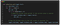Selection Sort implemented using Python

Assume line-wise time complexity and number of times executed is as follows;

`line 4    --> C1 --> n-1 timesline 7,8  --> C2 --> x timesline 11   --> C3 --> n-1 times`

Here, the x times can be explained as

`x = (n-1)+(n-2)+(n-3)+ ... + 1 = n(n-1)/2`

In the execution of this method, total time complexity will sum up to:

`T(n) =  C1(n-1) + C2(n(n-1)/2) + C3(n-1)`

The simplification of the above equation will result in `an² + bn + c` type equation. Hence, the highest order of term is n². Therefore, time complexity of the algorithm is considered as O(n²) in Big O notation.

# Bubble Sort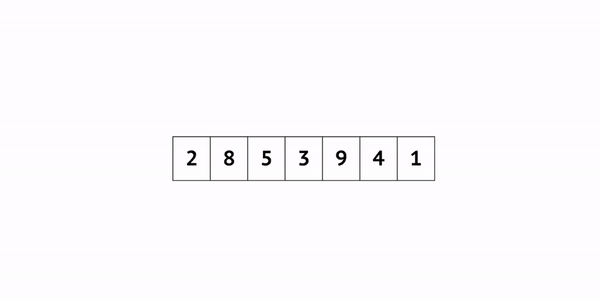Bubble Sort (Source: Michael Sambol — Youtube)

Compare two adjacent elements and swap if the left one is greater than the right one. Traverse the whole list for a single iteration. This will end up carrying the maximum value to the rightmost corner of the unsorted portion list, making it as the starting point of the sorted portion of the list.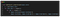Unoptimized version of Bubble Sort implemented using Python

The time complexity of the above algorithm can be calculated in a similar fashion to the Selection sort we discussed. It will also result in a `an² + bn + c` type equation. Hence, the time complexity is again O(n²).

However, in line 4, if you look clearly, you can see that we are looping again through the sorted portion of the list. We can optimize this part to loop through only the unsorted portion by using `n-iter_num-1` instead of `n-1`.

Another thing to consider is, if we have an iteration without any swap, that indicates the fact the list is already sorted. Hence, we don't need to loop through further iterations. We can use a flag to check this condition and stop looping.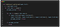Optimized version of Bubble Sort implemented using Python

However, still, the time complexity will remain same for worst or average cases. For the best-case scenario, (i.e. sorted in the first pass) time complexity will be O(n).

# Insertion Sort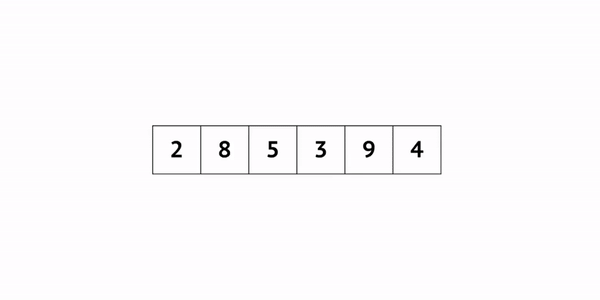Insertion Sort (Source: Michael Sambol — Youtube)

In Insertion sort, we start with the second element of the list, assuming that the first element is the sorted portion of the list. We compare the second element (key item) with the first element and swap if the key item is smaller than the first element. This will end up giving us 2 sorted elements to the left of the list. Then we consider the first element of the unsorted portion (i.e. third element → key item) and compare it with the rightmost corner of the sorted portion. If the key item is smaller, we swap the values. Then compare the key item with the value before it, and swap if the key item is smaller. Likewise, do this till the previous one is not larger than the key item or, till the key item reaches the leftmost corner of the sorted portion. Iterate through the whole list to complete sorting.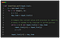Insertion Sort implemented using Python

Assume line-wise time complexity and number of times executed is as follows;

`line 4,5    --> C1 --> n-1 timesline 10,11  --> C2 --> n(n-1)/2 timesline 13     --> C3 --> n-1 times`

In the best-case scenario, line 10 & 11 will not execute. Hence time complexity will be:

`T(n) =  C1(n-1) + C3(n-1)T(n) => an + bT(n) => O(n)`

In the average or worst-case scenario, all the lines will be executed. Hence time complexity will be:

`T(n) =  C1(n-1) + C2(n(n-1)/2) + C3(n-1)T(n) => an² + bn + cT(n) => O(n²)`

However, number of comparisons and shifts in the Insertion sort is lesser than that of Selection or Bubble sort. Hence, considered better than those two.

# Merge Sort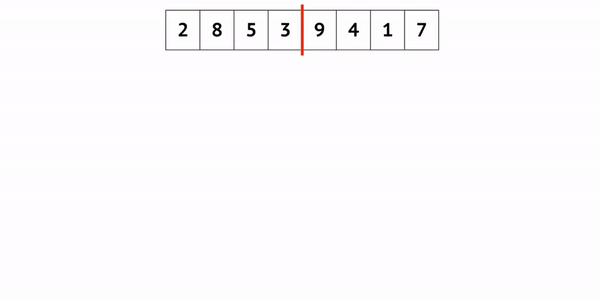Merge Sort (Source: Michael Sambol — Youtube)

Merge sort is different from the algorithms we have discussed up to now. It is a divide-and-conquer style algorithm, which is recursive and not in-place. It takes the list and divide recursively to the element level and merge back keeping the order.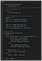Merge Sort implemented using Python

The time complexity of the Merge sort is O(n log n). Since it is not an in-place algorithm, it also has a space complexity of O(n).

# Quick Sort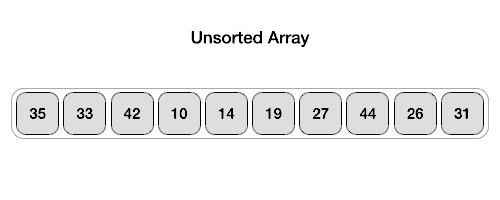Quick Sort (Source: Javaguides)

Quick Sort algorithm is another recursive algorithm for sorting. However, unlike Merge sort, this is a in-place sorting algorithm.

Sorting is started off by selecting a pivot (Selecting this pivot properly contributes a lot to the efficiency of the algorithm). Pivot is simply one element in the list. However, after sorting for one pass, it should meet following three conditions:

1. It is in the correct position of the final sorted list
2. Items to the left of the pivot are smaller
3. Items to the right of the pivot are larger

After selecting a pivot, we traverse the list from two corners of the list and compare the left selected item with the pivot and the right selected item with the pivot. If the left one is larger than the pivot, it becomes a candidate for a swap. If the right is one is smaller than pivot it becomes a candidate for a swap. If not we move the pointer towards the middle, till we find a possible candidate for a swap. When we find possible two candidates to swap, we swap those two elements. We do this till the pointers meet at the middle, and pivot is swapped to its correct position in the middle. This process repeats again and again by selecting different pivots, until the list is fully sorted.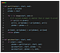Quick Sort implemented using Python

In the Quicksort algorithm, the average time complexity is O(n log n) while the worst-case scenario is O(n²).

As mentioned before, choosing a pivot is the challenge in this sorting algorithm. Choosing a randomize value as a pivot (rather than choosing starting or ending element as the pivot), almost all the time avoids the worst-case scenario. Therefore, most of the implementations of the Quicksort uses randomize value as the pivot. There is also another popular method called median-of-three. In this method, start, middle and end elements of the array is considered. We sort these 3 and choose the middle one as the pivot. The assumption here is that, the chosen pivot is closer to the median of the entire list.

# Summary of main sorting algorithms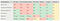Summary of main sorting algorithms with respect to time complexity, space complexity, stability and recursiveness.

Hope you learnt something new from today’s post. I tried my best to keep it sort and sweet 😅 Criticisms and Questions are warmly welcomed. Let’s meet in another post soon. 💪🏽

Happy Coding! ❤️

## The Startup

Get smarter at building your thing. Join The Startup’s +785K followers.

### By The Startup

Get smarter at building your thing. Subscribe to receive The Startup's top 10 most read stories — delivered straight into your inbox, once a week. Take a look.

By signing up, you will create a Medium account if you don’t already have one. Review our Privacy Policy for more information about our privacy practices.

Check your inbox
Medium sent you an email at to complete your subscription.

Written by

## Savindi Wijenayaka

ML Engineer & Researcher interested in contributing to products that cater to Healthcare; self-learner & love to share knowledge; https://phantomgrin.github.io/

## The Startup

Get smarter at building your thing. Follow to join The Startup’s +8 million monthly readers & +785K followers.

Written by

## Savindi Wijenayaka

ML Engineer & Researcher interested in contributing to products that cater to Healthcare; self-learner & love to share knowledge; https://phantomgrin.github.io/## The Startup

Get smarter at building your thing. Follow to join The Startup’s +8 million monthly readers & +785K followers.

Medium is an open platform where 170 million readers come to find insightful and dynamic thinking. Here, expert and undiscovered voices alike dive into the heart of any topic and bring new ideas to the surface. Learn more

Follow the writers, publications, and topics that matter to you, and you’ll see them on your homepage and in your inbox. Explore

If you have a story to tell, knowledge to share, or a perspective to offer — welcome home. It’s easy and free to post your thinking on any topic. Write on Medium